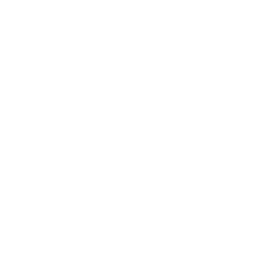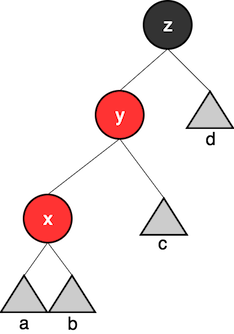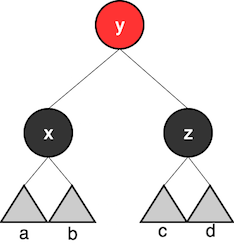Noel Markham (47 Degrees)## Hello## Agenda

• Brief introduction
• A brand new collection type

## Algebraic Data Types

#### What is an Algebraic Data Type?

According to Wikipedia:

In computer programming, especially functional programming and type theory, an algebraic data type is a kind of composite type, i.e., a type formed by combining other types.

Not to be confused with abstract data types.

## Counting Values

How many different values can these types have:

• `Boolean`?
• `Integer`?
• `Unit`?
• `String`? `List`?

## Counting Values

• `(Boolean, Int)`?
• `Either[Boolean, Int]`?

Tuples are products

Eithers are coproducts

Take a look at Jon Pretty's Talk from flatMap(Oslo) in 2014

Aside: what about function Bool to Int?

## Counting Values

`(Boolean, Int)`

``case class Invoice(paid: Boolean, price: Int)``

Are they different?

## Counting Values

`Either[(Boolean, Int), String]`

``````sealed trait DatabaseResponse
case class Invoice(paid: Boolean, price: Int) extends DatabaseResponse
case class ErrorMessage(description: String) extends DatabaseResponse``````

Is this different to the Either?

## Counting Values

• Grouping of types, such as tuples or case classes, are referred to as products
• A choice of type, such as an Either, is referred to as a coproduct or sum type

Other languages, such as Haskell, make this distinction much clearer:

``data Suit = Club | Diamond | Heart | Spade``

## Some common types

#### Option

``````sealed abstract class Option[+A]{
//...
}
final case class Some[+A](value: A) extends Option[A]
//...
}
case object None extends Option[Nothing] {
//...
}``````

## Some common types

#### List

``````sealed abstract class List[+A] {
//...
}``````
``````final case class ::[B](val head: B, var tl: List[B]) extends List[B] {
//...
}``````
``````case object Nil extends List[Nothing] {
//...
}``````

## Some common types

#### JSON

We can encode the different types of field allowed in JSON:

• Number
• Boolean
• String
• Array
• JSON object
• Null

## Some common types

#### JSON

``````sealed trait Json
case class JsonNumber(d: Double) extends Json
case class JsonBoolean(b: Boolean) extends Json
case class JsonString(s: String) extends Json
case class JsonArray(l: List[Json]) extends Json
case class JsonObject(j: Json) extends Json
case object JsonNull extends Json``````

Top tip: Take a look at Circe for a nice implementation

## What's the value of this?

• Types are good
• A more intuituve and natural encoding of a domain than bare, "primitive" types
• We have a way to encode all possible states, with help from the compiler

• An aside: we can make our `Invoice(Boolean, Int)` type by replacing the `Boolean` with an ADT
• Perhaps something like:
``````sealed trait PaidStatus
case object Paid extends PaidStatus
case object AwaitingPayment extends PaidStatus``````

## What's the value of this?

• We can provide a very safe way to map our ADT on to any other type
• This is usually called folding

## Folding

`DatabaseResponse => A`

There are (at least) a couple of ways we can do this:

• Good old pattern matching
• We can build the functionality into our type
``````sealed trait DatabaseResponse {
def fold(fi: (Boolean, Int) => A, fe: String => A): A =
if(isSuccess) fi(this.paid, this.price) else fe(this.description)
}``````

## Folding

#### Folding Options

``````sealed abstract class Option[+A] {
//...

final def fold[B](ifEmpty: => B)(f: A => B): B =
if (isEmpty) ifEmpty else f(this.get)

//...
}``````
``def map[B](f: A => B): Option[B] = fold(None)(a => Some(f(a)))``
``def foreach(f: A => Unit): Unit = fold(())(a => f(a))``
``def get: B = fold(throw new NoSuchElementException("None.get"))(identity)``

## Folding

#### Folding JSON

``````sealed trait Json {

def fold[A](
jsonNumber: Double => A,
jsonBoolean: Boolean => A,
jsonString: String => A,
jsonArray: List[Json] => A,
jsonObject: Json => A,
jsonNull: => A): A = {
//...
}
}``````

## Folding

#### What's the point?

• We already have pattern matching
• Most folds probably use pattern matching under the hood anyway

## Folding

#### What's the point?

Imagine we add a new class to our DatabaseResponse:

``case class PurchaseRequest(item: Item, cost: Int) extends DatabaseResponse``

We diligently update our fold:

``````def fold
(fi: (Boolean, Int) => A,
fp: (Item, Int) => A,
fe: String => A): A = ...``````
• Top tip: Use folding where possible
• But turn on compiler warnings as errors
• This will help when you non-exhaustively pattern match
(amongst other things)

#### Red-Black Trees

From Wikipedia:

A red–black tree is a kind of self-balancing binary search tree. Each node of the binary tree has an extra bit, and that bit is often interpreted as the color (red or black) of the node. These color bits are used to ensure the tree remains approximately balanced during insertions and deletions.

#### Red-Black Trees

From Purely Functional Data Structures (Chris Okasaki):

• Invariant 1: No red node has a red child
• Invariant 2: Every path from the root to an empty node contains the same number of black nodes

#### Red-Black Trees

``````sealed trait Colour[+A]
case class R[A](a: A) extends Colour[A]
case class B[A](a: A) extends Colour[A]``````
``````sealed trait Tree[+A]
case object E extends Tree[Nothing]
case class T[A](l: Tree[A], elem: Colour[A], r: Tree[A]) extends Tree[A]``````

#### Red-Black Trees

Folding the colour

``````sealed trait Colour[+A] {
def fold[X](fr: A => X, fb: A => X): X =
if(this.isRed) fr(this.a) else fb(this.a)
}``````

And add a helper to the trait:

``def elem: A = fold(identity, identity)``

#### Useful operations

Is a given element in the tree?

``````def member[A](element: A, tree: Tree[A])(implicit oa: Ordering[A]):Boolean =
tree match {``````
``  case E => false``
``````  case T(l, c, r) =>
import oa._
val e = c.elem
if (element < e) member(element, l)
else if (element > e) member(element, r)
else true
}``````

#### Red-Black Trees

Inserting an element into the tree

``````def insert[A](element: A, tree: Tree[A])(implicit oa: Ordering[A]): Tree[A] = {

def ins(t: Tree[A]): Tree[A] =
t match {``````
``      case E => T(E, R(element), E)``
``````      case T(l, ee, r) =>
import oa._
val e = ee.elem
if (element < e) balance(ins(l), ee, r)
else if (element > e) balance(l, ee, ins(r))
else t
}``````
``````   ins(tree)
}``````

#### Red-Black Trees

Balancing the tree

We need to turn trees that look like this:#### Red-Black Trees

Balancing the tree

Into this:• Invariant 1: No red node has a red child
• Invariant 2: Every path from the root to an empty node contains the same number of black nodes

#### Red-Black Trees

``````def balance[A](ll: Tree[A], ee: Colour[A], rr: Tree[A]): Tree[A] =
(ll, ee, rr) match {``````
``````    case (T(T(a, R(x), b), R(y), c), B(z), d) =>
T(T(a, B(x), b), R(y), T(c, B(z), d))``````#### Red-Black Trees

``````def balance[A](ll: Tree[A], ee: Colour[A], rr: Tree[A]): Tree[A] =
(ll, ee, rr) match {``````
``````    case (T(T(a, R(x), b), R(y), c), B(z), d) =>
T(T(a, B(x), b), R(y), T(c, B(z), d))``````
``````   case (T(a, R(x), T(b, R(y), c)), B(z), d) =>
T(T(a, B(x), b), R(y), T(c, B(z), d))``````
``````    case (a, B(x), T(T(b, R(y), c), R(z), d)) =>
T(T(a, B(x), b), R(y), T(c, B(z), d))``````
``````    case (a, B(x), T(b, R(y), T(c, R(z), d))) =>
T(T(a, B(x), b), R(y), T(c, B(z), d))``````
``````    case _ => T(ll, ee, rr)
}``````